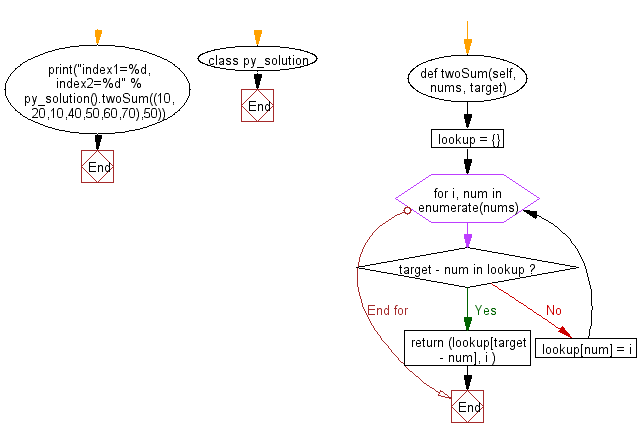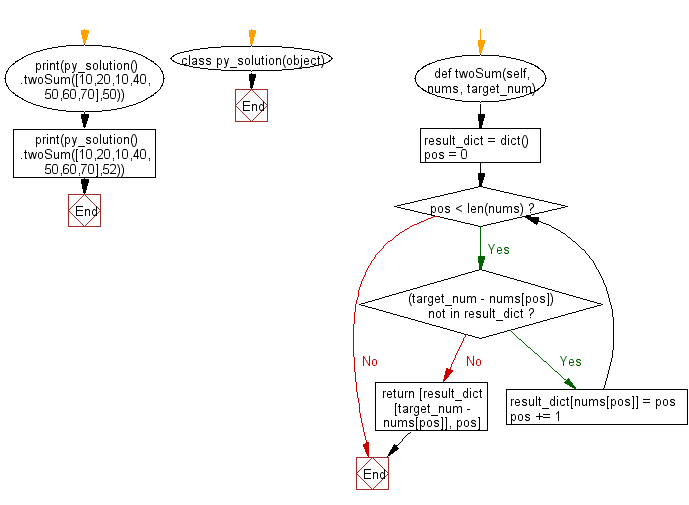﻿ Python: Find a pair of elements from a given array whose sum equals a specific target number - w3resource# Python: Find a pair of elements from a given array whose sum equals a specific target number

## Python Class: Exercise-5 with Solution

Write a Python class to find a pair of elements (indices of the two numbers) from a given array whose sum equals a specific target number.

Sample Solution-1:

Python Code:

``````class py_solution:
def twoSum(self, nums, target):
lookup = {}
for i, num in enumerate(nums):
if target - num in lookup:
return (lookup[target - num], i )
lookup[num] = i
print("index1=%d, index2=%d" % py_solution().twoSum((10,20,10,40,50,60,70),50))
```
```

Sample Output:

```index1=2, index2=3
```

Pictorial Presentation:Flowchart:## Visualize Python code execution:

The following tool visualize what the computer is doing step-by-step as it executes the said program:

Sample Solution-2:

Python Code:

``````class py_solution(object):
def twoSum(self, nums, target_num):
"""
:type nums: list[int]
:type target: int
:return type: list[int]
"""
result_dict = dict()
pos = 0
while pos < len(nums):
if (target_num - nums[pos]) not in result_dict:
result_dict[nums[pos]] = pos
pos += 1
else:
return [result_dict[target_num - nums[pos]], pos]
print(py_solution().twoSum([10,20,10,40,50,60,70],50))
print(py_solution().twoSum([10,20,10,40,50,60,70],52))
```
```

Sample Output:

```[2, 3]
None
```

Flowchart:## Visualize Python code execution:

The following tool visualize what the computer is doing step-by-step as it executes the said program:

Python Code Editor:

What is the difficulty level of this exercise?

Test your Programming skills with w3resource's quiz.

﻿

## Python: Tips of the Day

The Zip() Function:

```>>> students = ('John', 'Mary', 'Mike')
>>> ages = (15, 17, 16)
>>> scores = (90, 88, 82, 17, 14)
>>> for student, age, score in zip(students, ages, scores):
...     print(f'{student}, age: {age}, score: {score}')
...
John, age: 15, score: 90
Mary, age: 17, score: 88
Mike, age: 16, score: 82
>>> zipped = zip(students, ages, scores)
>>> a, b, c = zip(*zipped)
>>> print(b)
(15, 17, 16)
```